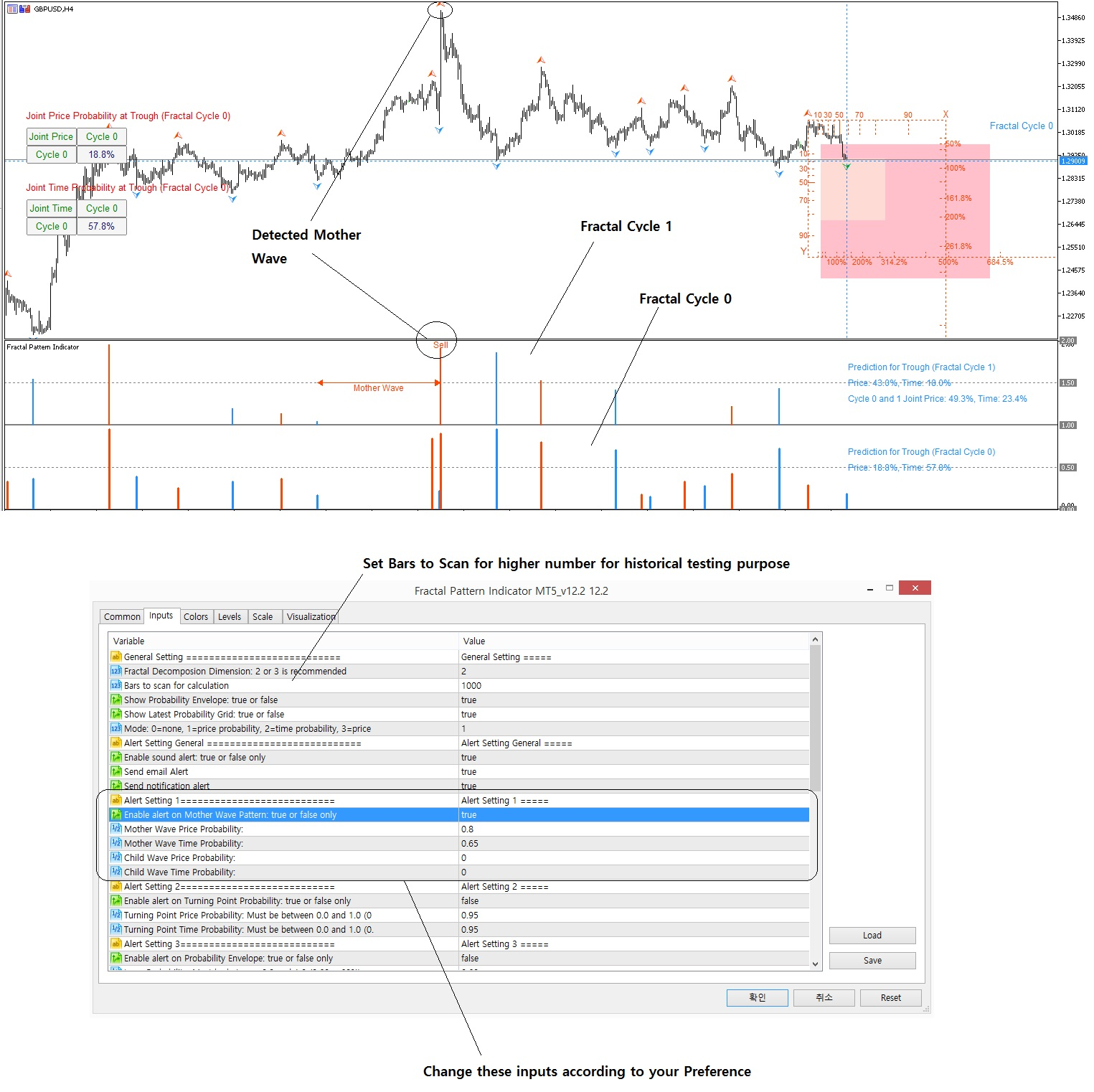# Mother Wave Detection Setting

In Fractal Pattern Scanner, we provide following features.

1. Probability of turning point in price and time for each peak and trough

2. Probability Envelope study to detect some extreme move (i.e. outlier)

3. Detecting Mother Wave with high chance of turning point probability

First of all, when mother wave is detected, it will be displayed in your indicator window like this. The inputs for mother wave detection is under Alert Setting 1.

You will see following inputs:

• Mother Wave Price Probability
• Mother Wave Time Probability
• Child Wave Price Probability
• Child Wave Time Probability

Considering you  are using two Fractal Cycles (i.e. Fractal Cycle 0 and Fractal Cycle 1), then Mother Wave Probability is simply the probability of bigger wave (i.e. Fractal Cycle 1). Child Wave probability is simply the probability of smaller wave (i.e. Fractal Cycle 0).

Hence, you have several different ways to control your Mother Wave detection. If you set any of above probability to zero, then it will be ignored. For example,

If you have inputs like this:

• Mother Wave Price Probability:  0.95 (95%)
• Mother Wave Time Probability:  0.50 (50%)
• Child Wave Price Probability:     0.0 (0%)
• Child Wave Time Probability:     0.0 (0%)

Then in Mother Wave detection, child wave probability will be not considered. Mother wave will be detected only if the Price probability and time probability of bigger wave is greater than 95% and 50% respectively.

On the other hand, you can have inputs like this:

• Mother Wave Price Probability:  0.50 (50%)
• Mother Wave Time Probability:  0.50 (50%)
• Child Wave Price Probability:     0.50 (50%)
• Child Wave Time Probability:     0.50 (50%)

In this Mother Wave detection setting, child wave probability must exceed 50% in price and time to be qualified as the mother wave for your signal.

To find the best setting works for your trading application, you can do little experiment. Typically, you will have few mother wave detected if you set probability too high. More mother wave will be detected if you set probability too low. However, these detected mother wave with lower probability might be too early before the market turning point.

Typically the detected mother wave will signal before the turning point. Hence, you might have to wait until actual turning point happens. After mother wave is detected, in your trading, you can use the provided Fractal Moving Average indicator and Fibonacci Retracement in price and time (i.e. in the Fibonacci-probability Grid) as secondary confirmation for your final decision. However, it is good to get alert earlier for the turning point happens than late because you can be ready for the big move before other trader.

Please understand the pros and cons of using high and low probability setting in detecting mother wave.

Here is the landing page to our  Fractal Moving Average Indicator (Free for everyone with some limitation for free users.)

https://www.mql5.com/en/market/product/46277

https://www.mql5.com/en/market/product/46276